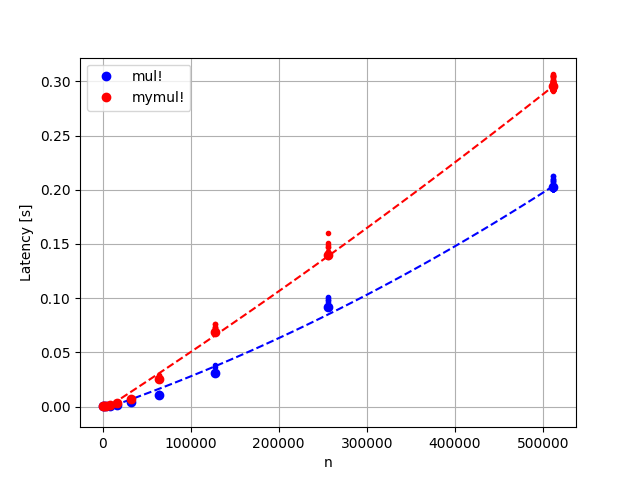# Non-linear latency of sparse-dense matrix multiplication

Hello,

I’m measuring the latency of `mul!(C, A, B)`, where `C` and `B` are dense and `A` is sparse, and I’m seeing something surprising: the latency isn’t linear in the number of operations required. Does anyone know why this is?

The number of columns of `A` is `m=1000`, the number of columns of `B` is `k=3`, and I’m varying the number of rows of `A`, denoted by `n`. I’m on an Intel system and I’m including `MKLSparse` (but I see the same behaviour regardless of if I use `MKLSparse`).

My experiment looks like the following code, except that it’s in a function, I’m running it many times, and I discard the first sample (since it includes compilation time)

``````A = sprand(n, m, 0.05)
B = randn(m, k)
C = zeros(n, k)
latency_mul = @elapsed mul!(C, A, B)
latency_mymul! = @elapsed mymul!(C, A, B) # mymul! defined below
``````

Here’s the definition of `mymul!`:

``````function mymul!(C::Matrix, A::SparseMatrixCSC, B::Matrix)
@boundscheck size(C, 1) == size(A, 1) || throw(DimensionMismatch("C has dimensions \$(size(C)), but A has dimensions \$(size(A))"))
@boundscheck size(C, 2) == size(B, 2) || throw(DimensionMismatch("C has dimensions \$(size(C)), but B has dimensions \$(size(B))"))
@boundscheck size(A, 2) == size(B, 1) || throw(DimensionMismatch("A has dimensions \$(size(A)), but B has dimensions \$(size(B))"))
m, n = size(A)
k = size(B, 2)
rows = rowvals(A)
vals = nonzeros(A)
C .= 0
for col = 1:n # column of A
for i in nzrange(A, col)
@inbounds row = rows[i] # row of A
@inbounds val = vals[i] # A[row, col]
@simd for j = 1:k # columns indices of B
@inbounds C[row, j] += val * B[col, j]
end
end
end
C
end
``````

This is the latency I’m seeing. I’d have expected that latency would increase linearly with the number of rows, which is what I’m seeing for `mymul!` (ish, for large enough matrices). However, for `mul!` I see something else. The dashed lines are quadratic functions fit to the data. The latency looks linear in the number of rows when running the same experiment for dense matrices so it’s got something to do with the sparse matrix multiplication.1 Like

What a coincidence! I just need an ad hoc implementation of subspace iteration, where one of the major costs is the multiplication of a beefy sparse matrix with a huge dense matrix of vectors as columns.
I guess my system is not as fast as yours, since in my case the execution time (which I think you call “latency”) is on the order of seconds. (Of course, it also depends on how sparse the matrix is. I know.)

I haven’t actually tried what you describe as an in-house solution. I rather tried using threads to calculate the individual columns. I did not get any speed up, unfortunately.

Isn’t the access to `C` row-major in your code?

Threading should ideally be handled by the matrix multiplication routines without you having to think about it. If you’re on an Intel system I’d recommend trying out `MKLSparse`. There’s some text suggesting it can speed up sparse matrix operations significantly when using multiple threads, see https://github.com/JuliaSparse/MKLSparse.jl/issues/24#issuecomment-658086827

The access to `C` is based on what I believe is the fastest way to iterate over the non-zero entries of `A`. I may be wrong though. On the other hand, I don’t really care about the `mymul!`. I’ve only implemented it as a reference.

I understand. Still, one wonders if it could be done faster.

I’m more interested in the non-linearity. There’s something I’m not understanding about how `mul!` is computed.

Is the sparsity changing as you increase the size?

No.
Everything is kept constant except the number of rows.

Does that mean the “sparsity”, or the average number of nonzeros per column?

Does it make a difference?I generate `A` like this:

``````A = sprand(n, 1000, 0.05)
``````

I suppose not that much. However the clustering of the non-zeros might.